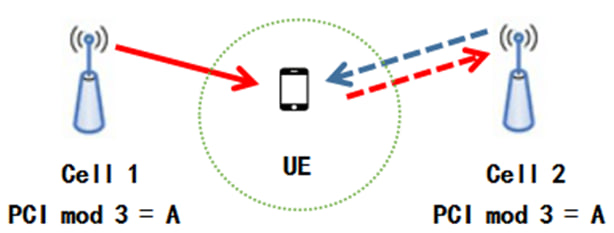# How to check PCI Mod 3 Conflicts with Excel

To check PCI (Physical Cell Identity) Mod 3 conflict for two cells in LTE using an Excel formula, you can follow these steps:

1. Prepare your data: Create an Excel spreadsheet and organize your data in columns, with each cell represented by its corresponding PCI.

2. Use a formula in Excel: In a separate column, you can use the following formula to check for PCI Mod 3 conflicts between two cells:

=MOD(ABS(A1-B1), 3)

Here, A1 and B1 represent the cell references for the two PCI values you want to compare.

1. Interpret the results: The formula will output a result between 0 and 2. If the result is 0, it means there is a PCI conflict under Mod 3. If the result is 1 or 2, there is no conflict.

Using this formula, you can easily identify whether there is a PCI Mod 3 conflict between two cells in LTE. You can apply the formula to multiple pairs of cells by dragging it down the column or extending the formula horizontally.